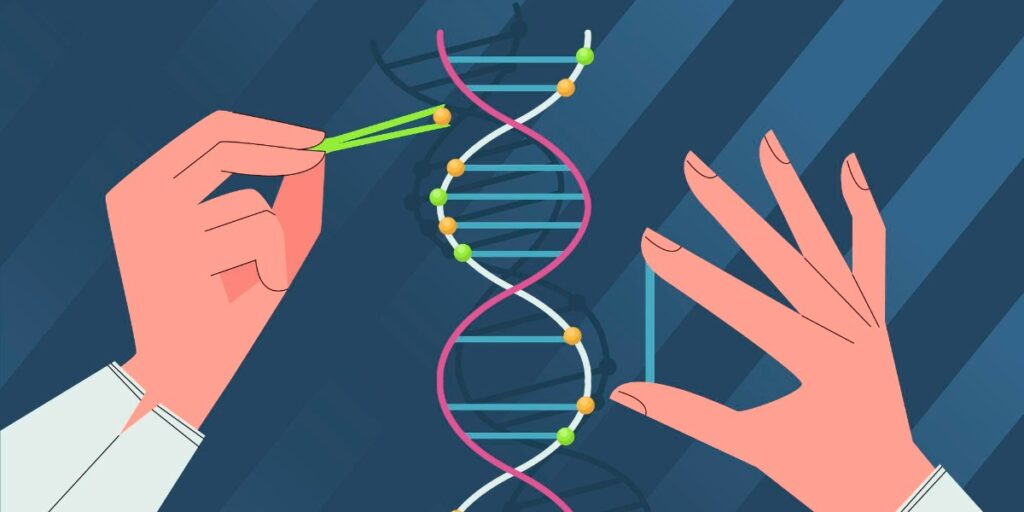# How To Calculate The Number Of Molecules In Any Piece of DNA

Posted in: Basic Lab Skills and Know-howYou can calculate the number of DNA molecules (per gram) in your DNA sample by:
multiplying the amount of DNA in ng by Avogadro’s number (6.022 x 10e23). This value is then divided by the product of [the DNA length in base pairs x (1 x10e9) x 650].

Lab math – it’s a dreaded topic, isn’t it? Calculating molecules in DNA samples, calculating the concentration of a solution, or working out serial dilutions were tasks that always filled me with dread as an undergrad. And to this day, I am still not a huge fan! Fear not – there is light at the end of the tunnel!

In this article, we will go through counting molecules in DNA samples – for instance, how many templates/copies of a 50-base pair (bp) fragment do you have in a 500 ng sample?

You will be a whiz at these calculations in no time!

## Calculating Molecules in DNA – Why Would I Need To?

This information may come in useful when doing the following types of experiments:

1. DNA ligations.
2. Biochemical assays.
3. Real-time PCR (absolute quantification).
4. Measuring viral load/numbers of viral genomes.

Whatever you need this information for, you can go about it in the same step-by-step fashion.

## Here Comes the Science…

• The average weight of a single DNA bp is 650 daltons. This can also be written as 650 g/mol (= molar mass).
• This is the same as saying that one mole of a bp weighs 650 g.
• The molecular weight or molar mass of any double-stranded DNA fragment can therefore be calculated by multiplying its length (in bp) by 650 and the answer will be expressed as daltons or g/mol.

As long as you know the sequence length (i.e. the length/size of the genome), this calculation will work for the genomic DNA of any species. To get the length of your sequence in bp, count the nucleotides in the DNA sequence – either by hand or using character count in a word processing program. If you need some help with converting your calculations between different units, check out our guide for solving your lab math problems.

Question: What is the molecular weight of a 5.2-kb plasmid? Remember 5.2 kb = 5200 bp

Calculation: 5200 bp x 650 daltons = 3,380,000 daltons or g/mol

Now we know how to calculate the molecular weight of a DNA template. Using Avogadro’s number, which is 6.022 x 1023 molecules/mole, the number of molecules of the template per gram can be calculated using the following formula:

$\textrm{Number of copies} = (\textup{\textrm{}ng} \times [6.022 \times 10^{23}])/(\textrm{length }\times [1 \times 10^{9}] \times 650)$

• ng is the amount of DNA (plasmid, primer, etc.) you have in nanograms.
• 6.022 x 1023 = Avogadro’s number.
• Length is the length of your DNA fragment in base pairs. Just multiply by 1000 if you are working in kb.
• We multiply by 1 x 109 to convert our answer to nanograms.

Note: the Avogadro’s number or constant (denoted as NA) is defined as the number of constituent particles (usually atoms or molecules) per mole of a given substance.

## Calculating Molecules in DNA: Useful Examples

### Example 1: Plasmid

Question: You have 300 ng of a 5.2-kb plasmid.

How many copies of your plasmid do you have?

Calculation: Number of copies =

$(300 \times [6.022 \times 10^{23}])/(5200 \times [1 \times 10^{9}] \times 650)$

### Example 2: Primer

Question: You have 50 ng of a 20-bp primer.

How many copies of your primer do you have?

Calculation: Number of copies =

$(50 \times [6.022 \times 10^{23}])/(20 \times [1 \times 10^{9}] \times 650)$

### Example 3: PCR product

Question: You have 150 ng of a 670-bp purified PCR product.

How many copies of your PCR product do you have?

Calculation: Number of copies =

$(150 \times [6.022 \times 10^{23}])/(670 \times [1 \times 10^{9}] \times 650)$

Note:  It’s important to remember that this formula is based on the assumption that you are working with a single DNA species, so when applying to PCR products or plasmids, make sure you only have 1 band or that you have a clean plasmid miniprep.

See, that wasn’t so bad after all. Once you know the length of your DNA fragment and the concentration in nanograms, you can easily apply the formula to get the number of copies in any type of DNA sample.

Want all your helpful DNA equations in one place in the lab? Download our free lab math cheat sheet for a handy formula and lots of helpful calculations.

Happy calculating molecules in DNA. If you have any comments, queries, or suggestions, get in touch using the comment box below.

Originally published September 17, 2014. Reviewed and updated April 27, 2021.

1.Anu on October 11, 2019 at 8:02 pm

The molecular weight (Da) of the zwitterionic form of the peptide GAGAGAGA?

2.fan xi wen on June 2, 2019 at 5:01 am

3.DATrevino on September 1, 2018 at 4:20 pm